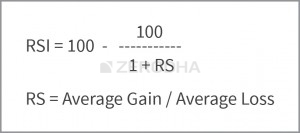# RSI Calculation in excel

Hi All here,
I am trying to calculate RSI in excel based on the calculation given in Zerodha Varsity Technical Analysis book.

@AlgoGeek Can you.

The formula for calculating the RSI value given in varsity & kite chartiq/tradingview is the same as given below.I have taken reliance 1-year daily OHLC data in excel and verified the RSI values, calculating with the above formula, It’s matching with kite tradingview/chartiq.

In varsity, the calculation is shown for the first RSI value with the simple average.
In excel calculation for RSI 14, we lookup avg of the first 14 gain/loss values and For the second RSI value we take( previous avg * 13 + present avg gain/loss ) /14, this gives like smoothed average value.

2 Likes

Hi @AlgoGeek,

Thank you so much for the details response, this will help a lot.

if the second part of the calculation is updated in varsity book, it will be helpful to those who want to give a try. do you have any idea to whom we need to request it.

You can put it as comment on that varsity page itself.

2 Likes

Thank you so much…

@siva, @AlgoGeek I just posted the solution, you have given for RSI problem in Varsity page.
Also updated my python program, now it works fine. Thanks for your help.

1 Like